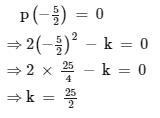# Test: Factorisation Factor Theorem

## 20 Questions MCQ Test Mathematics (Maths) Class 9 | Test: Factorisation Factor Theorem

Description
This mock test of Test: Factorisation Factor Theorem for Class 9 helps you for every Class 9 entrance exam. This contains 20 Multiple Choice Questions for Class 9 Test: Factorisation Factor Theorem (mcq) to study with solutions a complete question bank. The solved questions answers in this Test: Factorisation Factor Theorem quiz give you a good mix of easy questions and tough questions. Class 9 students definitely take this Test: Factorisation Factor Theorem exercise for a better result in the exam. You can find other Test: Factorisation Factor Theorem extra questions, long questions & short questions for Class 9 on EduRev as well by searching above.
QUESTION: 1

### x-a is a factor of p(x) = ax2+bx+c. Which of the following is true?​

Solution:

See if g(x) = x- a
Then g(x) is a factor of p(x)
The zero of polynomial = a
Therefore p(a)= 0

QUESTION: 2

### If x+1 is a factor of x3+3x2+3x+a, then a = ?

Solution:

Given, x3 + 3x2 + 3x + a = 0

and its factor is x + 1 = 0

⇒ x = -1

Put the value in equation i.e. x3 + 3x2 + 3x + a = 0

it becomes = (-1) + 3 (1) + 3(-1) + a = 0

⇒ -1 + 3 - 3 + a = 0

⇒ a = 1

QUESTION: 3

### If (x-2) is the factor of x2 + 2x+ a, find the value of ‘a’.

Solution:

If x-2 is a factor then 2 is the zero of the polynomial
by substituting 2 in the given polynomial,we get
2^2+2(2)+a=0
8+a=0
a=-8

QUESTION: 4

If a polynomial p(x) is divided by a linear divisor (x-a), then the remainder is

Solution:
QUESTION: 5

The value of p for which x + p is a factor of x2 + px + 3 – p is:

Solution:
QUESTION: 6

For two polynomials p(x) and q(x), x-a and x-b are their factors, respectively.Which of the following is true?​

Solution:
QUESTION: 7

Find the value of a such that (x – 2) is the factor of the polynomial x4 + ax3 + 2x2– 3x

Solution:

Zero of the polynomial is x -2 =0 =>x = 2 put the value of x = 2 (2²)² + a(2)³ + 2(2)² - 3(2) = 16 + 8a + 8 - 6 = 18 + 8a = 0 = 8a = -18 = a = -18/8 = a = -9/4

QUESTION: 8

One of the linear factors of ​3x2 + 8x + 5 is

Solution:
QUESTION: 9

What is the value of b such that x+3 is a factor of x2 - bx -12?

Solution:
QUESTION: 10

Find the value of ‘a’ so that (x-1) is the factor of x2+a+1

Solution:
QUESTION: 11

For a polynomial p(x), x-2 is a factor, so p(2) is _______​

Solution:
QUESTION: 12

x+1 is a factor of p(x) = x3+3x2+3x+1, so what is the value of p(-1)

Solution:
QUESTION: 13

If (x-1) is a factor of ax – a, then find the value of a​

Solution:

Solution :- f(x) = ax - a……………………..(1)

x - 1 = 0

x = 1

Putting in eq(1)

(1)x - 1 => x - 1

For eg :- if we put x = 100

Putting in eq(1)

(100)x - 100 => x - 100

So, all the values of a

QUESTION: 14

For a polynomial p(x), p(-1) and p(2) are both equal to zero .So, we can conclude that,

Solution:
QUESTION: 15

For a polynomial, x2 – 3x +2, which of the following is a factor ?

Solution:
QUESTION: 16

If (2x + 5) is a factor of 2x2 – k, then the value of k is.

Solution:

Let p(x) = 2x2 − k
Since, (2x+5) is a factor of p(x), then by factor
theorem,QUESTION: 17

(x + 2) is a factor of 2x3 + 5x2 - x - k. The value of k is:

Solution:
QUESTION: 18

If x-2 is a factor of ax2-x-6, so what should be the value of a?

Solution:
QUESTION: 19

Find the value of p(2) if p(x) has (x-2) as its factor

Solution:
QUESTION: 20

Which of the following polynomials has -3 as a zero?

Solution: